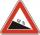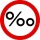# Road

Between cities A and B is route 13 km long of stúpanie average 7‰. Calculate the height difference of cities A and B.

Result

h =  91 m

#### Solution:Leave us a comment of example and its solution (i.e. if it is still somewhat unclear...):

Showing 0 comments:Be the first to comment!#### To solve this example are needed these knowledge from mathematics:

Our permille calculator will help you quickly calculate various typical tasks with permilles. Do you want to convert length units? See also our right triangle calculator. See also our trigonometric triangle calculator. Try conversion angle units angle degrees, minutes, seconds, radians, grads.

## Next similar examples:

1. Height differenceWhat height difference overcome if we pass road 1 km long with a pitch21 per mille?
2. Descent of roadRoad sign informs the gradient is 10.3%. Calculate the angle which average decreases.
3. Slope of trackCalculate the average slope (in promiles and even in degrees) of the rail tracks between Prievidza (309 m AMSL) and Topoľčany (174 m AMSL), if the track is 44 km long.
4. Two municipalitiesThe horizontal distance between municipalities is 39 km. Average sinking is 7 per mille. What is the difference in height between these municipalities?
5. 30-60-90The longer leg of a 30°-60°-90° triangle measures 5. What is the length of the shorter leg?
6. Height 2Calculate the height of the equilateral triangle with side 38.
7. SinesIn ▵ ABC, if sin(α)=0.5 and sin(β)=0.6 calculate sin(γ)
8. MapleMaple peak is visible from a distance 3 m from the trunk from a height of 1.8 m at angle 62°. Determine the height of the maple.
9. LiftThe largest angle at which the lift rises is 16°31'. Give climb angle in permille.
10. ReflectorCircular reflector throws light cone with a vertex angle 49° and is on 33 m height tower. The axis of the light beam has with the axis of the tower angle 30°. What is the maximum length of the illuminated horizontal plane?
11. Reference angleFind the reference angle of each angle:
12. Center traverseIt is true that the middle traverse bisects the triangle?
13. TrigonometryIs true equality? ?
14. High wallI have a wall 2m high. I need a 15 degree angle (upward) to second wall 4 meters away. How high must the second wall?
15. RailwayRailway line had on 5.8 km segment climb 9 permille. How many meters track ascent?
16. TreeHow tall is the tree that observed in the visual angle of 52°? If I stand 5 m from the tree and eyes are two meters above the ground.
17. Theorem proveWe want to prove the sentense: If the natural number n is divisible by six, then n is divisible by three. From what assumption we started?